# Image Transmission and Reception Using LTE Waveform and SDR

This example shows how to transmit and receive an image using an LTE waveform and a software-defined radio (SDR). The example generates a single or multi-antenna LTE waveform for simultaneous transmission and reception on a single SDR platform. During waveform generation, the example encodes and packs an image into a radio frame. Then, on reception, the example decodes the image from the received LTE waveform.

### IntroductionThe LTE Toolbox™ generates standard-compliant baseband IQ downlink and uplink reference measurement channel (RMC) waveforms and downlink test model (E-TM) waveforms. Using an SDR such as the Xilinx® Zynq®-Based Radio, you can modulate these waveforms for RF transmission and reception.

This example imports an image file and packs it into multiple radio frames of a baseband RMC waveform generated by using the LTE Toolbox. The example creates a continuous RF LTE waveform by using the repeated transmission functionality of a supported SDR. The repeated transmission functionality transfers the baseband RMC waveform to the hardware memory on the SDR and transmits the waveform continuously over the air without gaps. If you use an SDR device capable of multiple channel transmission and reception, the example generates and transmits a multi-antenna LTE waveform using LTE Transmit Diversity.

The example captures the resultant waveform by using the same SDR. If you have the appropriate hardware, the example uses multi-channel reception in the receiver.

This example supports these SDRs.

• USRP™ E310/E312 from the Communications Toolbox Support Package for USRP™ Embedded Series Radio

• AD936x/FMCOMMS5 from the Communications Toolbox Support Package for Xilinx® Zynq®-Based Radio

Alternatively, if an SDR is not available, the example supports simulating an additive white gaussian noise (AWGN) channel or passing the waveform directly to the decode stage with no impairments.

### Example Setup

Before running the example, set the `channel` variable to one of these options:

• `OverTheAir`: Use an SDR to transmit and receive the WLAN waveform

• `GaussianNoise`: Pass the transmission waveform through an AWGN channel (default)

• `NoImpairments`: Pass the transmission waveform through with no impairments

`channel ="GaussianNoise";`

If you set `channel` to `OverTheAir`, configure the transmit gain and center frequency, and set `deviceName` to the desired SDR:

• Set to `Pluto` to use the ADALM-Pluto Radio (default)

• Set to `E3xx` to use the USRP Embedded Series Radio

• Set to `AD936x` or `FMCOMMS5` to use the Xilinx Zynq-Based Radio

If you set channel to `GaussianNoise`, set the signal-to-noise ratio (SNR) for the receive waveform.

```if channel == "OverTheAir" deviceName =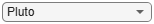"Pluto"; centerFrequency =2200000000; txGain =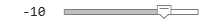-10; elseif channel == "GaussianNoise" % Specify SNR of received signal for a simulated channel SNR =20; end```

Configure these parameters for LTE waveform generation.

```txsim.RC ='R.7'; % Base RMC configuration, 10 MHz bandwidth txsim.NCellID =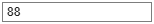88; % Cell identity txsim.NFrame =700; % Initial frame number txsim.NumAntennas =1; % Number of transmit and receive antennas```

Configure all the scopes and figures for the example.

Set up a handle for the image plot.

```if ~exist("imFig", "var") || ~ishandle(imFig) imFig = figure; imFig.NumberTitle = "off"; imFig.Name = "Image Plot"; imFig.Visible = "off"; else clf(imFig); % Clear figure imFig.Visible = "off"; end```

Set up a handle for the channel estimate plots.

```if ~exist("hhest", "var") || ~ishandle(hhest) hhest = figure("Visible","Off"); hhest.NumberTitle = "off"; hhest.Name = "Channel Estimate"; else clf(hhest); % Clear figure hhest.Visible = "off"; end```

Set up a spectrum scope to view the received waveform later in the example.

```spectrumScope = spectrumAnalyzer( ... SpectrumType="power-density", ... Title="Received Baseband LTE Signal Spectrum", ... YLabel="Power spectral density", ... ShowLegend=true);```

Set up the constellation diagram viewer for the equalized physical downlink shared channel (PDSCH) symbols.

```constellation = comm.ConstellationDiagram(Title="Equalized PDSCH Symbols", ... ShowReferenceConstellation=false);```

Determine and set the positions for all figures in this example.

```[positionsValid,positions] = hPlotPositions; if positionsValid imFig.Position = positions(5,:); hhest.Position = positions(1,:); spectrumScope.Position = positions(6,:); constellation.Position = positions(3,:); end```

### Transmitter Design: System Architecture

The general structure of the LTE transmitter consists of these steps:

1. Import and convert an image to a binary data stream.

2. Generate a baseband LTE signal using LTE Toolbox, packing the binary data stream into the transport blocks of the downlink shared channel (DL-SCH).

3. If using an SDR, prepare the baseband signal for transmission using the SDR hardware and send the baseband data to the SDR for continuous transmission at the desired center frequency.

Prepare Image File

The example reads data from the image file, scales it for transmission, and converts it to a binary data stream. The size of the transmitted image directly impacts the number of LTE radio frames required for the transmission of the image data. A scaling factor of `0.5` requires five LTE radio frame transmissions. Increasing the scaling factor results in more frame transmissions. Reducing the scaling factor reduces the number of frames.

Specify the image file name and its scaling factor.

```fileTx ='peppers.png'; % Image file name scale =0.5; % Image scaling factor```

Import the specified image file and covert it to a binary stream.

```fData = imread(fileTx); % Read image data from file origSize = size(fData); % Original input image size scaledSize = max(floor(scale.*origSize(1:2)),1); % Calculate new image size heightIx = min(round(((1:scaledSize(1))-0.5)./scale+0.5),origSize(1)); widthIx = min(round(((1:scaledSize(2))-0.5)./scale+0.5),origSize(2)); fData = fData(heightIx,widthIx,:); % Resize image imsize = size(fData); % Store new image size binData = dec2bin(fData(:),8); % Convert to 8 bit unsigned binary trData = reshape((binData-'0').',1,[]).'; % Create binary stream```

Using the previously created handle for the image plot, display the transmit image. Later in the example, if the LTE waveform is successfully decoded, the received image displays beneath the transmitted image.

```% Plot transmit image figure(imFig); imFig.Visible = "on"; subplot(211); imshow(fData); title("Transmitted Image"); subplot(212); title("Received image will appear here..."); set(gca,"Visible","off"); % Hide axes```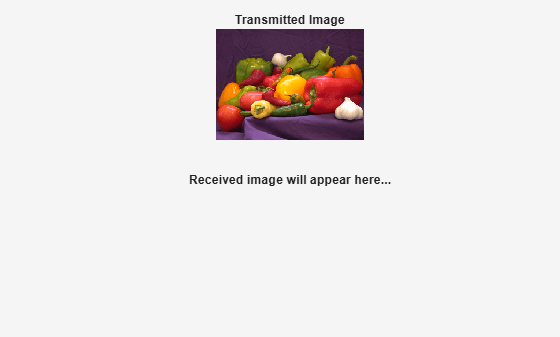`set(findall(gca, "type", "text"), "visible", "on"); % Unhide title`

Generate Baseband LTE Signal

Use the `lteRMCDL` (LTE Toolbox) function to generate the default configuration parameters for an RMC as defined in TS36.101 Annex A.3 [ 1 ]. The parameters within the configuration structure `rmc` allow customization as required.

`rmc = lteRMCDL(txsim.RC);`

Calculate the required number of LTE frames based on the size of the image data.

```trBlkSize = rmc.PDSCH.TrBlkSizes; txsim.TotFrames = ceil(numel(trData)/sum(trBlkSize(:)));```

Customize RMC parameters

```rmc.NCellID = txsim.NCellID; rmc.NFrame = txsim.NFrame; rmc.TotSubframes = txsim.TotFrames*10; % 10 subframes per frame rmc.CellRefP = txsim.NumAntennas; % Configure number of cell reference ports rmc.PDSCH.RVSeq = 0;```

Fill subframe 5 with dummy data.

```rmc.OCNGPDSCHEnable = "On"; rmc.OCNGPDCCHEnable = "On";```

If transmitting over two or more channels, enable transmit diversity.

```if rmc.CellRefP >= 2 rmc.PDSCH.TxScheme = "TxDiversity"; rmc.OCNGPDSCH.TxScheme = "TxDiversity"; else rmc.PDSCH.TxScheme = "Port0"; rmc.OCNGPDSCH.TxScheme = "Port0"; end rmc.PDSCH.NLayers = txsim.NumAntennas; fprintf("\nGenerating LTE transmit waveform:\n"+ ... " Packing image data into %d frame(s).\n\n", txsim.TotFrames);```
```Generating LTE transmit waveform: Packing image data into 5 frame(s). ```

Use the `lteRMCDLTool` (LTE Toolbox) to generate a baseband waveform, `eNodeBOutput`, a fully populated resource grid, `txGrid`, and the full configuration of the RMC. The tool takes the binary stream, `trData`, created from the input image file and packs it into multiple transport blocks in the PDSCH.

```% Pack the image data into a single LTE frame [eNodeBOutput,txGrid,rmc] = lteRMCDLTool(rmc,trData);```

Transmit LTE Waveform

If using an SDR, create an `sdrTransmitter` object using the `sdrtx` function. Set the center frequency, sample rate, gain, and channel configuration to the corresponding properties of the `sdrTransmitter` object. Then, use the `transmitRepeat` function to transfer the baseband LTE transmission to the SDR platform for continuous transmission.

```if channel == "OverTheAir" % Transmitter properties sdrTransmitter = sdrtx(deviceName); sdrTransmitter.BasebandSampleRate = rmc.SamplingRate; % 15.36 MHz for default RMC (R.7) with a bandwidth of 10 MHz sdrTransmitter.CenterFrequency = centerFrequency; sdrTransmitter.Gain = txGain; sdrTransmitter.ChannelMapping = 1:txsim.NumAntennas; % Pass the SDR I/O directly to host skipping FPGA on Zynq Radio or USRP % Embedded Series Radio if deviceName ~= "Pluto" sdrTransmitter.ShowAdvancedProperties = true; sdrTransmitter.BypassUserLogic = true; end fprintf("\nGenerating LTE transmit waveform:\n") % Scale the normalized signal to avoid saturation of RF stages powerScaleFactor = 0.8; eNodeBOutput = eNodeBOutput.*(1./max(abs(eNodeBOutput))*powerScaleFactor); % Transmit RF waveform transmitRepeat(sdrTransmitter,eNodeBOutput); end```

The general structure of the LTE receiver consists of these steps:

1. If using an SDR, capture a suitable number of frames of the transmitted LTE signal. Otherwise, apply AWGN to the `eNodeBOutput` or apply no impairments.

2. Determine and correct the frequency offset of the received signal.

3. Synchronize the captured signal to the start of an LTE frame.

4. OFDM demodulate the received signal to get an LTE resource grid.

5. Perform a channel estimation for the received signal.

6. Decode the PDSCH and DL-SCH to obtain the transmitted data from the transport blocks of each radio frame.

This example plots the power spectral density of the captured waveform, and shows visualizations of the estimated channel, equalized PDSCH symbols, and received image.

Capture one more LTE frame than transmitted to allow for timing offset wraparound in receiver processing.

```framesPerCapture = txsim.TotFrames+1; % Number of LTE frames to capture. captureTime = framesPerCapture * 10e-3; % Capture time in seconds```

Create an SDR receiver System Object with the specified properties for the device used for the image transmission. The sample rate of the receiver is 15.36MHz, which is the standard sample rate for capturing an LTE bandwidth of 50 resource blocks (RBs). 50 RBs is equivalent to a signal bandwidth of 10 MHz.

```if channel == "OverTheAir" sdrReceiver = sdrrx(deviceName); sdrReceiver.BasebandSampleRate = sdrTransmitter.BasebandSampleRate; sdrReceiver.CenterFrequency = sdrTransmitter.CenterFrequency; sdrReceiver.OutputDataType = "double"; sdrReceiver.ChannelMapping = 1:txsim.NumAntennas; if deviceName ~= "Pluto" sdrReceiver.ShowAdvancedProperties = true; sdrReceiver.BypassUserLogic = true; end fprintf("\nStarting a new RF capture.\n") rxWaveform = capture(sdrReceiver,captureTime,"Seconds"); elseif channel == "GaussianNoise" rxWaveform = awgn(eNodeBOutput,SNR,"measured"); else % No Impairments rxWaveform = eNodeBOutput; end```

Show the power spectral density of the captured waveform.

```spectrumScope.SampleRate = rmc.SamplingRate; spectrumScope(rxWaveform); release(spectrumScope)```The example simplifies the LTE signal reception by assuming that the transmitted PDSCH properties are known.

Assume FDD duplexing mode and a normal cyclic prefix length, as well as four cell-specific reference ports (CellRefP) for the master information block (MIB) decode. The MIB provides the number of actual CellRefP.

```enb.PDSCH = rmc.PDSCH; enb.DuplexMode = "FDD"; enb.CyclicPrefix = "Normal"; enb.CellRefP = 4;```

The sampling rate of the signal controls the captured bandwidth. Obtain the number of RBs captured from a lookup table using the chosen sampling rate.

```% Bandwidth: {1.4 3 5 10 20 } MHz SampleRateLUT = [1.92 3.84 7.68 15.36 30.72]*1e6; NDLRBLUT = [6 15 25 50 100]; enb.NDLRB = NDLRBLUT(SampleRateLUT==rmc.SamplingRate); if isempty(enb.NDLRB) error("Sampling rate not supported. Supported rates are %s.",... "1.92 MHz, 3.84 MHz, 7.68 MHz, 15.36 MHz, 30.72 MHz"); end```

Configure channel estimation to be performed by using cell-specific reference signals. A 9-by-9 averaging window minimizes the effect of noise.

```% Channel estimation configuration structure cec.PilotAverage = "UserDefined"; % Type of pilot symbol averaging cec.FreqWindow = 9; % Frequency window size in REs cec.TimeWindow = 9; % Time window size in REs cec.InterpType = "Cubic"; % 2D interpolation type cec.InterpWindow = "Centered"; % Interpolation window type cec.InterpWinSize = 3; % Interpolation window size```

Process Captured Signal

As the LTE waveform is continuously transmitted over the air in a loop, the first frame captured by the receiver is not guaranteed to be the first transmitted frame. This means that the frames may be decoded out of sequence. To enable the received frames to be recombined in the correct order, their frame numbers must be determined. The MIB contains information on the current system frame number, and therefore must be decoded. After the frame number has been determined, the example decodes the PDSCH and DL-SCH and displays the equalized PDSCH symbols. No data is transmitted in subframe 5; therefore, the captured data for subframe is ignored for the decoding.

When the LTE frames have been successfully decoded, the example displays the detected frame number on a frame-by-frame basis and the equalized PDSCH symbol constellation for each subframe. The example also displays an estimate of the channel magnitude frequency response between cell reference point 0 and the receive antenna for each frame.

Perform a frequency offset correction for known cell ID.

```frequencyOffset = lteFrequencyOffset(enb,rxWaveform); rxWaveform = lteFrequencyCorrect(enb,rxWaveform,frequencyOffset); fprintf("\nCorrected a frequency offset of %i Hz.\n",frequencyOffset)```
```Corrected a frequency offset of 2.473473e-01 Hz. ```

Perform the blind cell search to obtain cell identity and timing offset. Use `"PostFFT"` SSS detection method to improve detection speed.

```cellSearch.SSSDetection = "PostFFT"; cellSearch.MaxCellCount = 1; [NCellID,frameOffset] = lteCellSearch(enb,rxWaveform,cellSearch); enb.NCellID = NCellID; fprintf("Detected a cell identity of %i.\n", NCellID);```
```Detected a cell identity of 88. ```

Sync the captured samples to the start of an LTE frame, and trim off any samples that are part of an incomplete frame.

```rxWaveform = rxWaveform(frameOffset+1:end,:); samplesPerFrame = 10e-3*rmc.SamplingRate; % LTE frames period is 10 ms tailSamples = mod(length(rxWaveform),samplesPerFrame); rxWaveform = rxWaveform(1:end-tailSamples,:); enb.NSubframe = 0; fprintf("Corrected a timing offset of %i samples.\n",frameOffset)```
```Corrected a timing offset of 0 samples. ```

OFDM demodulate the waveform and perform channel estimation for 4 cell-specific reference ports as the cell-specific reference ports are unknown for the eNodeB.

```rxGrid = lteOFDMDemodulate(enb,rxWaveform); [hest,nest] = lteDLChannelEstimate(enb,cec,rxGrid); sfDims = lteResourceGridSize(enb); Lsf = sfDims(2); % OFDM symbols per subframe LFrame = 10*Lsf; % OFDM symbols per frame numFullFrames = length(rxWaveform)/samplesPerFrame;```

Initialize these variables for processing the received frames.

```rxDataFrame = zeros(sum(enb.PDSCH.TrBlkSizes(:)),numFullFrames); recFrames = zeros(numFullFrames,1); rxSymbols = []; txSymbols = [];```

For each frame, decode the MIB, PDSCH, and DL-SCH.

```for frame = 0:(numFullFrames-1) fprintf("\nPerforming DL-SCH Decode for frame %i of %i in burst:\n", ... frame+1,numFullFrames) % Extract subframe #0 from each frame of the received resource grid % and channel estimate. enb.NSubframe = 0; rxsf = rxGrid(:,frame*LFrame+(1:Lsf),:); hestsf = hest(:,frame*LFrame+(1:Lsf),:,:); % PBCH demodulation. Extract resource elements (REs) % corresponding to the PBCH from the received grid and channel % estimate grid for demodulation. enb.CellRefP = 4; pbchIndices = ltePBCHIndices(enb); [pbchRx,pbchHest] = lteExtractResources(pbchIndices,rxsf,hestsf); [~,~,nfmod4,mib,CellRefP] = ltePBCHDecode(enb,pbchRx,pbchHest,nest); % If PBCH decoding successful CellRefP~=0 then update info if ~CellRefP fprintf(" No PBCH detected for frame.\n"); continue; end enb.CellRefP = CellRefP; % Decode the MIB to get current frame number enb = lteMIB(mib,enb); % Incorporate the nfmod4 value output from the function % ltePBCHDecode, as the NFrame value established from the MIB % is the system frame number modulo 4. enb.NFrame = enb.NFrame+nfmod4; fprintf(" Successful MIB Decode.\n") fprintf(" Frame number: %d.\n",enb.NFrame); % Store received frame number recFrames(frame+1) = enb.NFrame; % Process subframes within frame (ignoring subframe 5) for sf = 0:9 if sf~=5 % Ignore subframe 5 % Extract subframe enb.NSubframe = sf; rxsf = rxGrid(:,frame*LFrame+sf*Lsf+(1:Lsf),:); % Perform channel estimation with the correct number of CellRefP [hestsf,nestsf] = lteDLChannelEstimate(enb,cec,rxsf); % Physical control format indicator channel (PCFICH) % demodulation. Extract REs corresponding to the PCFICH from % the received grid and channel estimate for demodulation. pcfichIndices = ltePCFICHIndices(enb); [pcfichRx,pcfichHest] = lteExtractResources(pcfichIndices,rxsf,hestsf); [cfiBits,recsym] = ltePCFICHDecode(enb,pcfichRx,pcfichHest,nestsf); % Control format indicator (CFI) decoding enb.CFI = lteCFIDecode(cfiBits); % Get PDSCH indices [pdschIndices,pdschIndicesInfo] = ltePDSCHIndices(enb, enb.PDSCH, enb.PDSCH.PRBSet); [pdschRx, pdschHest] = lteExtractResources(pdschIndices, rxsf, hestsf); % Perform deprecoding, layer demapping, demodulation and % descrambling on the received data using the estimate of % the channel [rxEncodedBits, rxEncodedSymb] = ltePDSCHDecode(enb,enb.PDSCH,pdschRx,... pdschHest,nestsf); % Append decoded symbol to stream rxSymbols = [rxSymbols; rxEncodedSymb{:}]; %#ok<AGROW> % Transport block sizes outLen = enb.PDSCH.TrBlkSizes(enb.NSubframe+1); % Decode DownLink Shared Channel (DL-SCH) [decbits{sf+1}, blkcrc(sf+1)] = lteDLSCHDecode(enb,enb.PDSCH,... outLen, rxEncodedBits); %#ok<SAGROW> % Recode transmitted PDSCH symbols for EVM calculation % Encode transmitted DLSCH txRecode = lteDLSCH(enb,enb.PDSCH,pdschIndicesInfo.G,decbits{sf+1}); % Modulate transmitted PDSCH txRemod = ltePDSCH(enb, enb.PDSCH, txRecode); % Decode transmitted PDSCH [~,refSymbols] = ltePDSCHDecode(enb, enb.PDSCH, txRemod); % Add encoded symbol to stream txSymbols = [txSymbols; refSymbols{:}]; %#ok<AGROW> constellation(rxEncodedSymb{:}); % Plot current constellation release(constellation); % Release previous constellation plot end end % Reassemble decoded bits fprintf(" Retrieving decoded transport block data.\n"); rxdata = []; for i = 1:length(decbits) if i~=6 % Ignore subframe 5 rxdata = [rxdata; decbits{i}{:}]; %#ok<AGROW> end end % Store data from receive frame rxDataFrame(:,frame+1) = rxdata; % Plot channel estimate between CellRefP 0 and the receive antennae focalFrameIdx = frame*LFrame+(1:LFrame); figure(hhest); hhest.Visible = "On"; surf(abs(hest(:,focalFrameIdx,1,1))); shading flat; xlabel("OFDM symbol index"); ylabel("Subcarrier index"); zlabel("Magnitude"); title("Estimate of Channel Magnitude Frequency Response"); end```
```Performing DL-SCH Decode for frame 1 of 5 in burst: ```
``` Successful MIB Decode. ```
``` Frame number: 700. ```
``` Retrieving decoded transport block data. ```
```Performing DL-SCH Decode for frame 2 of 5 in burst: ```
``` Successful MIB Decode. ```
``` Frame number: 701. ```
``` Retrieving decoded transport block data. ```
```Performing DL-SCH Decode for frame 3 of 5 in burst: ```
``` Successful MIB Decode. ```
``` Frame number: 702. ```
``` Retrieving decoded transport block data. ```
```Performing DL-SCH Decode for frame 4 of 5 in burst: ```
``` Successful MIB Decode. ```
``` Frame number: 703. ```
``` Retrieving decoded transport block data. ```
```Performing DL-SCH Decode for frame 5 of 5 in burst: ```
``` Successful MIB Decode. ```
``` Frame number: 704. ```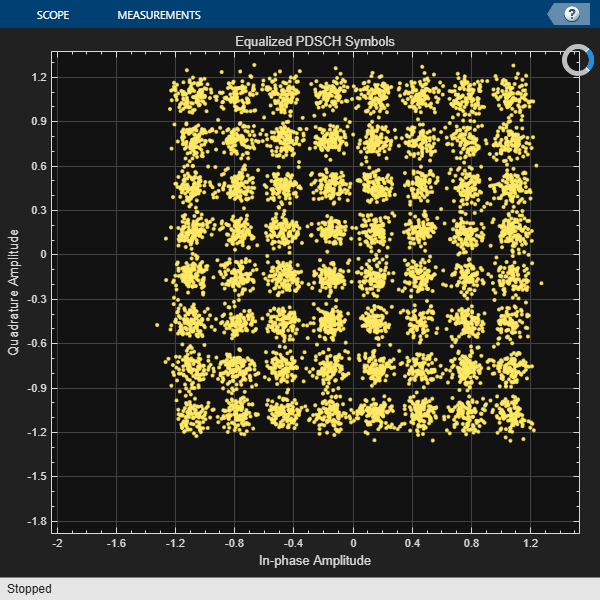``` Retrieving decoded transport block data. ``````if channel == "OverTheAir" release(sdrTransmitter); release(sdrReceiver); end```

Result Qualification and Display

To determine the quality of the received data, the example calculates the bit error rate (BER) between the transmitted and received data. The received data reforms into an image.

Determine the index of first transmitted frame (lowest received frame number).

`[~,frameIdx] = min(recFrames);`

Initialize these variables for image reconstruction.

```decodedRxDataStream = zeros(length(rxDataFrame(:)),1); frameLen = size(rxDataFrame,1);```

Recombine the received data blocks (in correct order) into a continuous stream.

```for n=1:numFullFrames currFrame = mod(frameIdx-1,numFullFrames)+1; % Get current frame index decodedRxDataStream((n-1)*frameLen+1:n*frameLen) = rxDataFrame(:,currFrame); frameIdx = frameIdx+1; % Increment frame index end```

Use the EVM and error rate System Objects, to calculate the EVM and bit error rate of the decoded data.

```if ~isempty(rxSymbols) evmCalculator = comm.EVM(); evmCalculator.MaximumEVMOutputPort = true; [evm.RMS,evm.Peak] = evmCalculator(txSymbols, rxSymbols); fprintf(" EVM peak = %0.3f%%\n",evm.Peak); fprintf(" EVM RMS = %0.3f%%\n",evm.RMS); % Perform bit error rate (BER) calculation bitErrorRate = comm.ErrorRate; err = bitErrorRate(decodedRxDataStream(1:length(trData)), trData); fprintf(" Bit Error Rate (BER) = %0.5f.\n",err(1)); fprintf(" Number of bit errors = %d.\n",err(2)); fprintf(" Number of transmitted bits = %d.\n",length(trData)); else fprintf(" No transport blocks decoded.\n"); end```
``` EVM peak = 32.669% ```
``` EVM RMS = 8.428% ```
``` Bit Error Rate (BER) = 0.00000. ```
``` Number of bit errors = 0. ```
``` Number of transmitted bits = 1179648. ```

Recreate the image from the received data.

```str = reshape(sprintf('%d',decodedRxDataStream(1:length(trData))), 8, []).'; decdata = uint8(bin2dec(str)); receivedImage = reshape(decdata,imsize); if exist("imFig", "var") && ishandle(imFig) % If TX figure is open figure(imFig); subplot(212); else figure; subplot(212); end imshow(receivedImage); title(sprintf("Received Image: %dx%d Antenna Configuration",txsim.NumAntennas, txsim.NumAntennas));```### Further Exploration

• If using an SDR, try reducing the transmitter gain to impair the quality of the received image and to increase the number of bit errors. Otherwise, reduce the signal-to-noise ratio (`SNR`).

• If using a supported multi-channel SDR, try increasing the number of antennas (`txsim.NumAntennas`) to visualize the benefit of using multi-channel transmission.

### Selected Bibliography

1. 3GPP TS 36.101. "User Equipment (UE) radio transmission and reception." 3rd Generation Partnership Project; Technical Specification Group Radio Access Network; Evolved Universal Terrestrial Radio Access (E-UTRA).